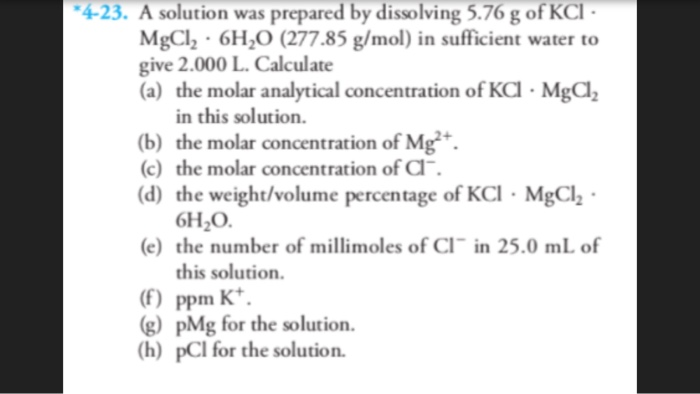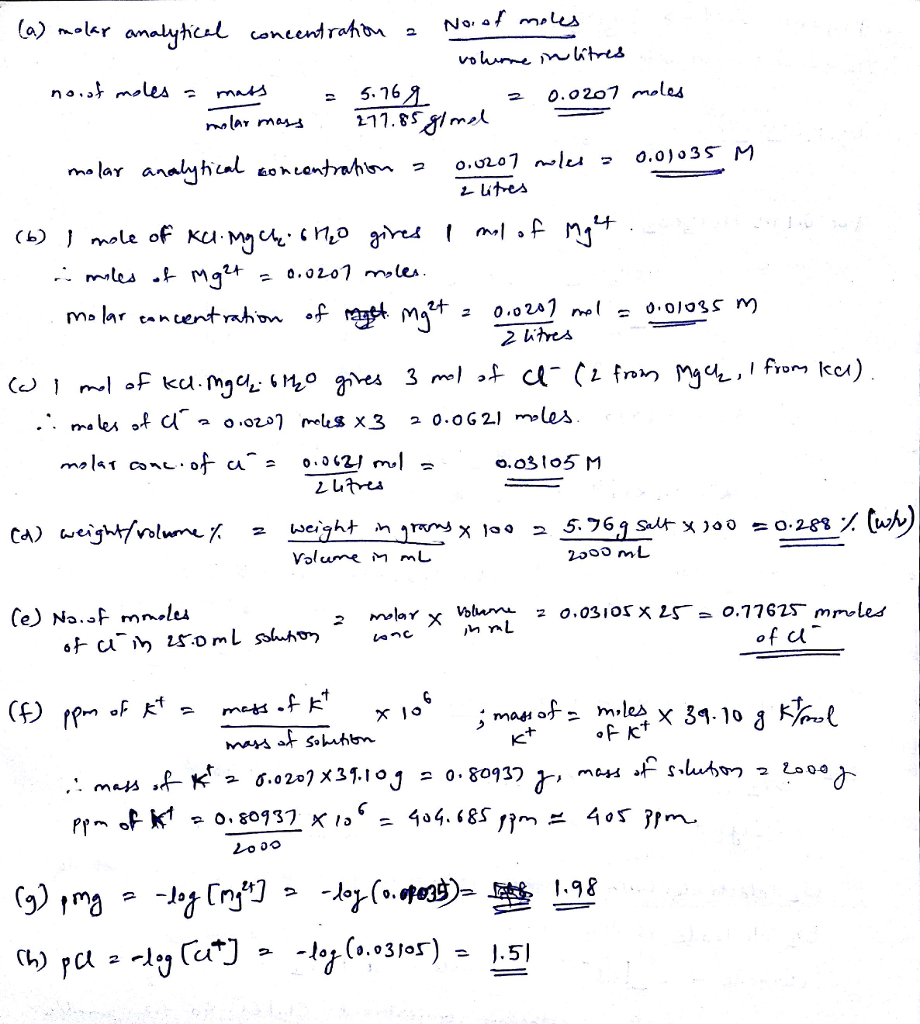# Question & Answer: A solution was prepared by dissolving 5.76 g of KCl. MgCl_2 middot 6H_2O (277.85…..A solution was prepared by dissolving 5.76 g of KCl. MgCl_2 middot 6H_2O (277.85 g/mol) in sufficient water to give 2.000 L. Calculate (a) the molar analytical concentration of KCl middot MgCl_2 in this solution. (b) the molar concentration of Mg^2+. (c) the molar concentration of Cl^-. (d) the weight/volume percentage of KCl middot MgCl_2 middot 6H_2O. (e) the number of millimoles of Cl^- in 25.0 mL of this solution (f) ppm K^+. (g) pMg for the solution. (h) pCl for the solution.